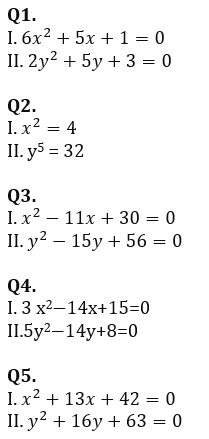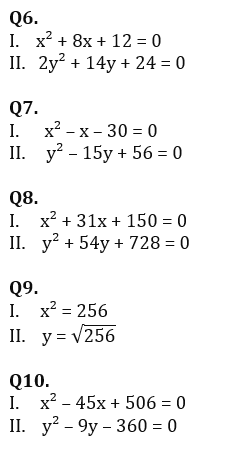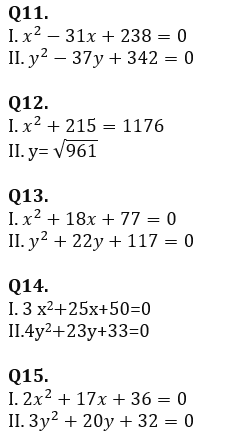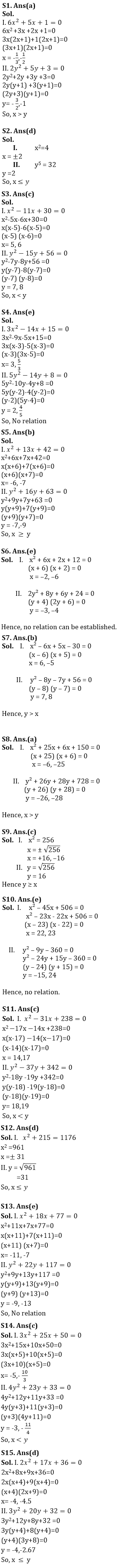Latest Banking jobs   »

# Quantitative Aptitude Quiz For Bank Foundation 2023 16th January

Directions (1-15): In each of the following questions, two equations (I) and (II) are given. Solve the equations and mark the correct option:
(a) if x > y
(b) if x ≥ y
(c) if x < y
(d) if x ≤ y
(e) if x = y or no relation can be established between x and y.Directions (6-10): Each of the following questions is provided with 2 statements i.e. Statement I & Statement II. You have to solve them and mark the correct option.
(a) x > y
(b) x < y
(c) x ≤ y
(d) x ≥ y
(e) x = y or no relation can be established.Directions (11-15): In each of the following questions, two equations (I) and (II) are given. Solve the equations and mark the correct option:
(a) if x > y
(b) if x ≥ y
(c) if x < y
(d) if x ≤ y
(e) if x = y or no relation can be established between x and y.Solutions.                                                                                                    .                                                                                                    .## FAQs

### When is the IBPS SO Mains exam scheduled to be held?

The IBPS SO Mains exam is scheduled to be held on 29th January 2023.

#### Congratulations!